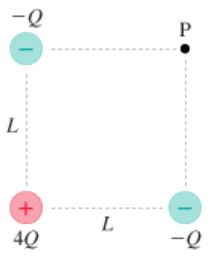# Problem: The figure(Figure 1) shows three charges at the corners of a square.Write the electric field at point P in component form. Assume that x-axis is horizontal and points to the right, and the y-axis points upward.Express your answer in terms of the variables Q, L, unit vectors i^, j^, and appropriate constants.

###### FREE Expert Solution

The electric field at a point is given by:

$\overline{){\mathbf{E}}{\mathbf{=}}\frac{\mathbf{k}\mathbf{q}}{{\mathbf{r}}^{\mathbf{2}}}}$, where is Coulomb's constant, q is charge., and r is the distance from the point to the charge.

The electric field at point P due to charge 1 (-Q).

$\begin{array}{rcl}{\mathbf{E}}_{\mathbf{1}\mathbf{x}}& \mathbf{=}& \frac{\mathbf{k}\mathbf{\left(}\mathbf{-}\mathbf{Q}\mathbf{\right)}}{{\mathbf{L}}^{\mathbf{2}}}\end{array}$

80% (391 ratings)###### Problem Details

The figure(Figure 1) shows three charges at the corners of a square.Write the electric field at point P in component form. Assume that x-axis is horizontal and points to the right, and the y-axis points upward.
Express your answer in terms of the variables Q, L, unit vectors i^, j^, and appropriate constants.